# Santander Case — Part A: Classification

## Here you will find: Data Cleaning, Feature Selection, Bayesian Optimization, Classification, and Model Validation.Customer Classification. Source: https://miro.medium.com/max/1400/1*PM4dqcAe6N7kWRpXKwgWag.png.

# The Problem

To be able to use this program properly, we need to develop a machine learning model to classify if the customer is satisfied or not. Customers classified as unsatisfied should be the target of the retention program.

The retention program cost \$10 for each customer and an effective application (in really unsatisfied customers) returns a profit of \$100. In the classification task we can have the following scenarios:

1. False Positive(FP): classify the customer as UNSATISFIED but he is SATISFIED. Cost: \$ 10, Earn: \$ 0;
2. False Negative(FN): classify the customer as SATISFIED but he is DISSATISFIED. Cost: \$ 0, Earn: \$ 0;
3. True Positive(TP): classify the customer as UNSATISFIED and he is UNSATISFIED. Cost: \$ 10, Earn: \$ 100;
4. True Negative(TN): classify the customer as SATISFIED and he is SATISFIED. Cost: \$ 0, Earn: \$ 0.

In summary, we want to minimize the rate of FP and FN as well as maximize the rate of TP. To do so, we will use the metric AUC (area under the curve) of ROC Curve (receiver operating characteristic), because it returns us the best model as well as the best threshold.

You can check the complete notebook with this solution on my Github.

This Case was made as a parte of tht prize for winning the Santander Data Masters Competition. I explain more about the competition itself and the hard skills I learned and soft skills I used in my way to winning it in this article.

Let’s go.

# 1 Loading Data and Packages

`# Loading packagesimport pandas as pdimport numpy as npimport matplotlib.pyplot as pltimport seaborn as snsimport time%matplotlib inline# Loading the Train and Test datasetsdf_train = pd.read_csv("data/train.csv")df_test = pd.read_csv("data/test.csv")`

The data can be found in this old Santanders Competition.

# 2 Basic Exploratory Analysis

• Are the data in the columns numeric or do they need to be encoded?
• Can the test dataset really be used or is it useful only for a Kaggle competition?
• Are there any missing data?
• What is the proportion of dissatisfied customers (1) in the dataset df_train?
• Does it make sense to apply a feature selection method on the data?

Primarily, let’s get a first overview of the dataset and its features

`# Checking the first 5 rows of df_traindf_train.head()`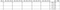df_train.head() output.
`# Checking the first 5 rows of df_testdf_test.head()`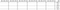df_test.head() output.
`# Checking the genearl infos of df_train and df_testdf_train.info()`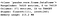df_train.info() output.
`# Checking the genearl infos of df_testdf_test.info()`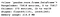df_test.info() output.

Looking at the outputs of the cells above, we can say that:

1. All columns are already in a numeric format. This means we don’t need to do any encoding to convert any type of variable into a numeric variable;
2. Since this is an anonymous dataset, we don’t have any clue if there are categorical variables. So, there is no need to make any encode to address this problem.
3. Lastly, df_train has 371 columns and df_test has 370 columns. This happens because as it comes from competitions datasets, the df_test has no Target column.

Another crucial point is to chek if there is any missing value on this datasets. Let’s check it out.

`# Checking if is there any missing value in both train and test datasetsdf_train.isnull().sum().sum(), df_test.isnull().sum().sum()`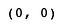No missing values for the datasets.

Now, we can conclude that there is no missing data in any dataset.

Finally, let’s investigate how is the proportion of unsatisfied customers (our target) in the df_train dataset.

`# Investigating the proportion of unsatisfied customers on df_trainrate_insatisfied = df_train.TARGET.value_counts() /                                            df_train.TARGET.value_counts()rate_insatisfied * 100`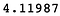Fraction of unsatisfied customers (%).

We have an extremely unbalanced dataset, approximately 4.12% positive. This must be taken into account in two situations:

1. To split the data in train and test;
2. To choose hyperparameters such as “class_weight” by Random Forest.

# 3 Dataset Split (train — test)

`from sklearn.model_selection import train_test_split# Spliting the dataset on a proportion of 80% for train and 20% for test.X_train, X_test, y_train, y_test = train_test_split(df_train.drop('TARGET', axis = 1), df_train.TARGET, train_size = 0.8, stratify = df_train.TARGET, random_state = 42)# Checking the splitX_train.shape, y_train.shape, X_test.shape, y_test.shape`

We split the test successfully in train data (X_train, y_train), and test data (X_test, y_test).

# 4 Feature Selection

1. To know which features bring most relevant prediction power to the model;
2. Avoid using features that could degrade the model performance;
3. Minimize the computational cost by using the minimal amount of features that provide the best model performance.

For this reason, we will try to answer the following questions:

• Are there any constant and/or semi-constants features that can be removed?
• Are there duplicate features?
• Does it make sense to perform some more filtering to reach a smaller group of features?

## 4.1 Removing low variance features

`# Investigating if there are constant or semi-constat feature in X_trainfrom sklearn.feature_selection import VarianceThreshold# Removing all features that have variance under 0.01selector = VarianceThreshold(threshold = 0.01)selector.fit(X_train)mask_clean = selector.get_support()X_train = X_train[X_train.columns[mask_clean]]`

Now let's check how much columns were removed.

`# Total of remaning featuresX_train.shape`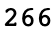Amount of remaining features.

With this filtering, 104 features were removed. Thus, the dataset has become leaner without losing predictive power. As these features do not add information to the machine learning model they impact its ability to classify an instance. We have now 266 features remaining.

## 4.2 Removing repeated features

`# Checking if there is any duplicated columnremove = []cols = X_train.columnsfor i in range(len(cols)-1):    column = X_train[cols[i]].values    for j in range(i+1,len(cols)):        if np.array_equal(column, X_train[cols[j]].values):            remove.append(cols[j])# If yes, than they will be dropped hereX_train.drop(remove, axis = 1, inplace=True)`

Now let’s check the result.

`# Checking if any column was droppedX_train.shape`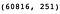The shape of X_train dataframe.

There were 266 columns before checking for duplicate features and now there are 251. So there were 15 repeated features.

## 4.3 Using SelectKBest to select features

Since the dataset is anonymized and the quantity of features is too large to make a quality study on the feature-target relationship, both methods will be tested and the one that produces a stable region with the highest AUC value will be chosen.

For this, different K values will be tested with the SelectKBest class, which will be used to train an XGBClassifier model and be evaluated using the AUC metric. Having a collection of values, a graph for fc and another for mic will be created.

Thus, through visual analysis, it is possible to choose the best K value as well as the best method for scoring features.

`from sklearn.feature_selection import SelectKBest, f_classif, mutual_info_classiffrom sklearn.metrics import roc_auc_score as aucfrom sklearn.model_selection import cross_val_scoreimport xgboost as xgb#Create an automated routine to test different K values in each of these methodsK_vs_score_fc = [] #List to store AUC of each K with f_classifK_vs_score_mic = [] #List to store AUC of each K with mutual_info_classifstart = time.time()for k in range(2, 247, 2):    start = time.time()        # Instantiating a KBest object for each of the metrics in order  to obtain the K features with the highest value    selector_fc = SelectKBest(score_func = f_classif, k = k)    selector_mic = SelectKBest(score_func = mutual_info_classif,     k = k)        # Selecting K-features and modifying the dataset    X_train_selected_fc = selector_fc.fit_transform(X_train,                                                                 y_train)    X_train_selected_mic = selector_mic.fit_transform(X_train, y_train)         # Instantiating an XGBClassifier object    clf = xgb.XGBClassifier(seed=42)        # Using 10-CV to calculate AUC for each K value avoinding  overfitting    auc_fc = cross_val_score(clf, X_train_selected_fc, y_train,     cv = 10, scoring = 'roc_auc')    auc_mic = cross_val_score(clf, X_train_selected_mic, y_train,    cv = 10, scoring = 'roc_auc')        # Adding the average values obtained in the CV for further  analysis.    K_vs_score_fc.append(auc_fc.mean())    K_vs_score_mic.append(auc_mic.mean())        end = time.time()    # Returning the metrics related to the tested K and the time   spent on this iteration of the loop    print("k = {} - auc_fc = {} - auc_mic = {} - Time =   {}s".format(k, auc_fc.mean(), auc_mic.mean(), end-start))        print(time.time() - start) # Computing the total time spent`

The code above returns two lists with 123 K-Scores. By plotting them for each value of K, we can choose the best K-value as well as the best features.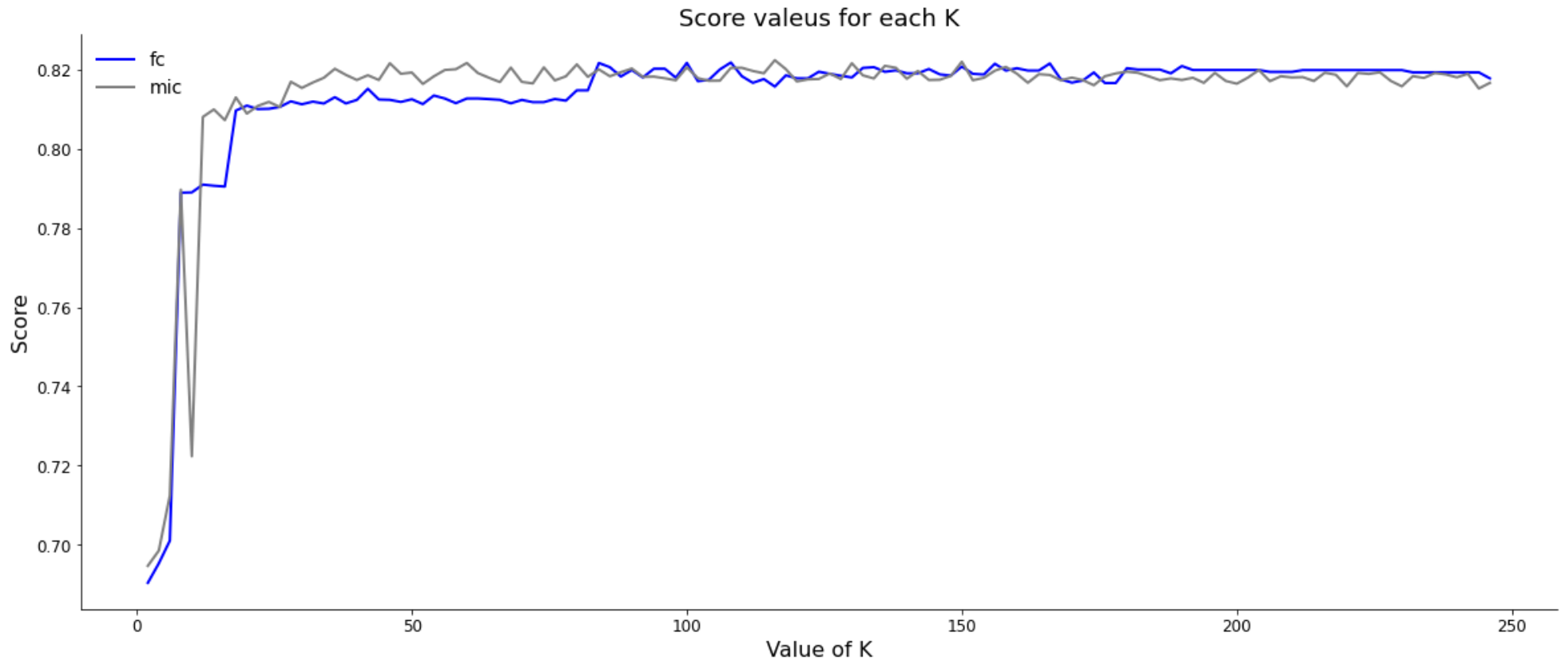Score values for both method (fc) and (mic).

Through the graphs above, it is noted that the best values are between 0.80 and 0.83 AUC. However, the graphs have a range from 0.70 to 0.83 due to small K values. To ensure a better evaluation of the K value and the method to be chosen for the next steps, let’s plot a visualisation for only the range between 0.80 and 0.83.

`# Ploting K_vs_score_fc e K_vs_score_mic (# of K-Best features vs AUC)import matplotlib.patches as patches# Figure setupfig, ax = plt.subplots(1, figsize = (20, 8))plt.title('Score valeus for each K', fontsize=18)plt.ylabel('Score', fontsize = 16)plt.xlabel('Value of K', fontsize = 16)ax.spines['top'].set_visible(False)ax.spines['right'].set_visible(False)plt.xticks(fontsize = 12)plt.yticks(fontsize = 12)# Create the linesplt.plot(np.arange(2, 247, 2), K_vs_score_fc, color='blue', linewidth=2)plt.plot(np.arange(2, 247, 2), K_vs_score_mic, color='grey', linewidth=2, alpha = 0.5)ax.legend(labels = ['fc', 'mic'], fontsize=14, frameon=False, loc = 'upper left')ax.set_ylim(0.80, 0.825);# Create a Rectangle patchrect = patches.Rectangle((82, 0.817), 20, (0.823 - 0.817), linewidth=2, edgecolor='r', facecolor='none')# Add the patch to the Axesax.add_patch(rect)plt.show()`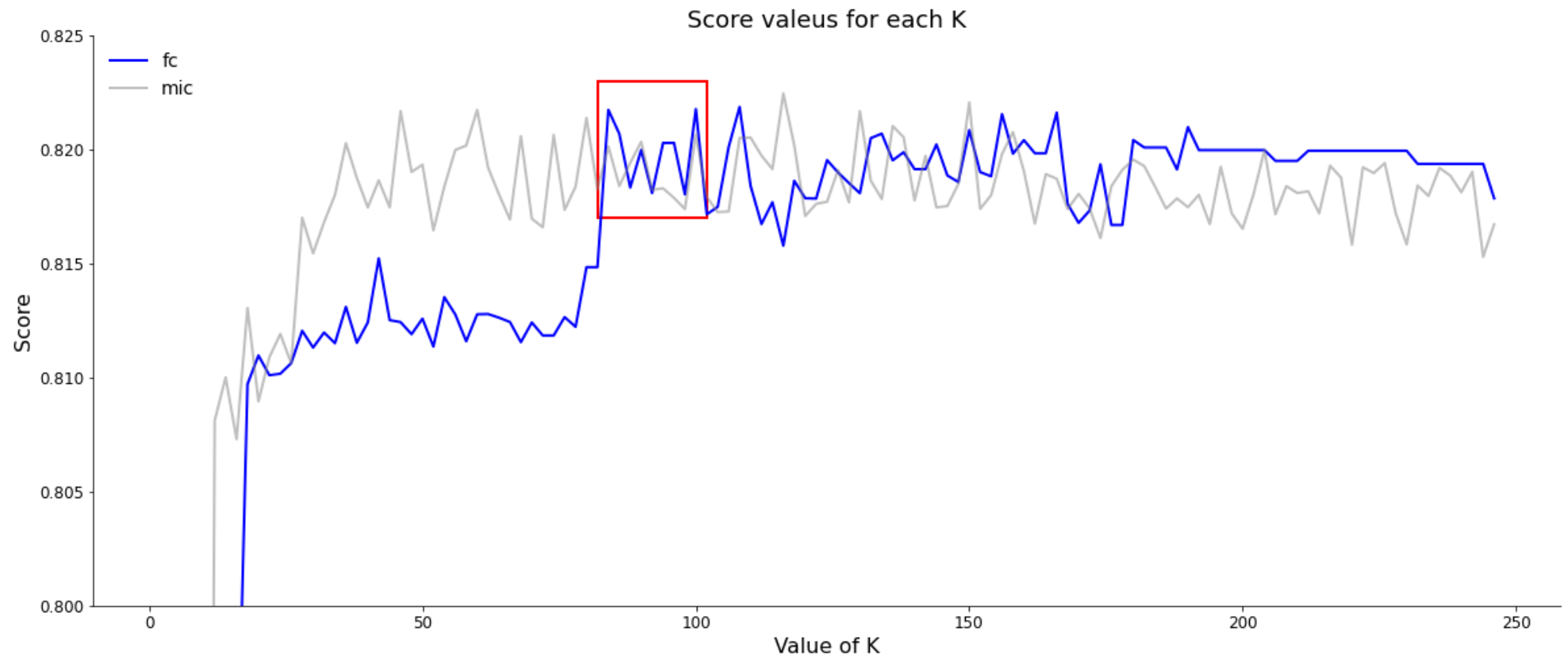Score values for both method (fc) and (mic) for the range 0,8 to 0,825.

By analyzing which method generates a better set of features to be used, we look for two main points:

• The smallest number of features that generate the highest AUC value;
• The region of the curve where the K-features are more stable because if K is just a peak, this can bring some instability to the model.

Applying these conditions to the above graphs, for fc and mic, we observed that although the mutual_info_classif method achieves slightly better results, it does not provide us any stable region.
Therefore, we selected for K the value of 96, which would be an intermediate point in this region.

In order to get a better understanding of the selected features, let’s visualize the bar chart for the first 30 best-scored features.

`# Ploting the score for the best 30 featuresfeature_score = pd.Series(selector_fc.scores_, index = X_train.columns).sort_values(ascending = False)fig, ax = plt.subplots(figsize=(20, 12))ax.barh(feature_score.index[0:30], feature_score[0:30])plt.gca().invert_yaxis()ax.set_xlabel('K-Score', fontsize=18);ax.set_ylabel('Features', fontsize=18);ax.set_title('30 best features by its K-Score', fontsize = 20)plt.yticks(fontsize = 14)plt.xticks(fontsize = 14)ax.spines['top'].set_visible(False)ax.spines['right'].set_visible(False)ax.spines['left'].set_visible(False);`

Now that we have the list of features that we will use for this task, we can create a dataset where only the desired features should be present.

`# Creating datasets where only the selected 96 features are includedX_train_selected = X_train[selected_col]X_test_selected = X_test[selected_col]`

Now that we have a good understanding of the features and a good selection of the ones who have the greatest impact on the model, we can move on to the next steps.

# 5 Bayesian Optimization to the XGBClassifier

So, by using an algorithm an interesting approach is to optimize its hyperparameters in a way we can have the best performance it can offer to us.

For this task, we will use the Bayesian Optimization approach. Some articles prove its greater efficiency when compared to grid search and a performance that is similar or even better than random search. Another advantage is that Bayesian Optimization allows us to optimize multiples hyperparameters at the same time.

`# Function for hyperparamters tunning# Implementation learned on a lesson of Mario Filho (Kagle Grandmaster) for parametes optmization.# Link to the video: https://www.youtube.com/watch?v=WhnkeasZNHIfrom skopt import forest_minimizedef tune_xgbc(params):    """Function to be passed as scikit-optimize minimizer/maximizer   input        Parameters:    Tuples with information about the range that the optimizer should use for that parameter,     as well as the behavior that it should follow in that range.        Returns:    float: the metric that should be minimized. If the objective is maximization, then the negative     of the desired metric must be returned. In this case, the negative AUC average generated by CV is returned.    """            #Hyperparameters to be optimized    print(params)    learning_rate = params     n_estimators = params     max_depth = params    min_child_weight = params    gamma = params    subsample = params    colsample_bytree = params                #Model to be optimized     mdl = xgb.XGBClassifier(learning_rate = learning_rate,                        .   n_estimators = n_estimators, max_depth = max_depth,     min_child_weight = min_child_weight, gamma = gamma,     subsample = subsample, colsample_bytree = colsample_bytree,     seed = 42)#Cross-Validation in order to avoid overfitting    auc = cross_val_score(mdl, X_train_selected, y_train,     cv = 10,    scoring = 'roc_auc')        print(auc.mean())    # as the function is minimization (forest_minimize), we need to use the negative of the desired metric (AUC)    return -auc.mean()`

Now let’s choose the hyperparameters intervals and call the function.

`# Creating a sample space in which the initial randomic search should be performedspace = [(1e-3, 1e-1, 'log-uniform'), # learning rate          (100, 2000), # n_estimators          (1, 10), # max_depth           (1, 6.), # min_child_weight           (0, 0.5), # gamma           (0.5, 1.), # subsample           (0.5, 1.)] # colsample_bytree# Minimization using a random forest with 20 random samples and 50 iterations for Bayesian optimization.result = forest_minimize(tune_xgbc, space, random_state=42, n_random_starts=20, n_calls=50, verbose=1)`

Now we can check the best hyperparameters found through the Bayesian Optimization.

`# Hyperparameters optimized valueshyperparameters = ['learning rate', 'n_estimators', 'max_depth', 'min_child_weight', 'gamma', 'subsample',                   'colsample_bytree']for i in range(0, len(result.x)):     print('{}: {}'.format(hyperparameters[i], result.x[i]))`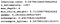Tunned hyperparameters through Bayesian Optimization.

To finish this part, we can also visualize the optimization’s convergence for the parameters that lead to the highest AUC.

In practice, the negative of the AUC was minimized, that is, the lower the value, the better the chosen parameters performed.

Thus, it is possible to notice some very relevant leaps along with the iterations of the Bayesian optimization. Near the sixth iteration, the negative of the AUC already signals that the hyperparameters found had reached a stable region and optimized values for the AUC.

Therefore, we proceed to the next steps with the optimal values found for the hyperparameters.

# 6 Model scoring

`# Generating the model with the optimized hyperparametersclf_optimized = xgb.XGBClassifier(learning_rate = result.x,   n_estimators = result.x, max_depth = result.x, min_child_weight = result.x, gamma = result.x, subsample = result.x, colsample_bytree = result.x, seed = 42)# Fitting the model to the X_train_selected datasetclf_optimized.fit(X_train_selected, y_train)`

Now that our model is tunned and trained, let’s test it on X_test_select, the part that we split from the training data in part 3.

`# Evaluating the performance of the model in the test data (which have not been used so far).y_predicted = clf_optimized.predict_proba(X_test_selected)[:,1]auc(y_test, y_predicted)`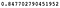Model AUC on X_test_select (Validation).

So, our model AUC Score was 0.8477. Pretty good so far!

Because the datasets come from an old Kaggle competition, we can validate the model’s performance on the test data (df_test) and score it on Kaggle website. Hereby, we can see what AUC value our model scores for 75818 instances that it has never seen before.

`# making predctions on the test dataset (df_test), from Kaggle, with the selected features and optimized parametersy_predicted_df_test = clf_optimized.predict_proba(df_test[selected_col])[:, 1]# saving the result into a csv file to be uploaded into Kaggle late subimission # https://www.kaggle.com/c/santander-customer-satisfaction/submitsub = pd.Series(y_predicted_df_test, index = df_test['ID'], name = 'TARGET')sub.to_csv('data/df_test_predictions.csv')`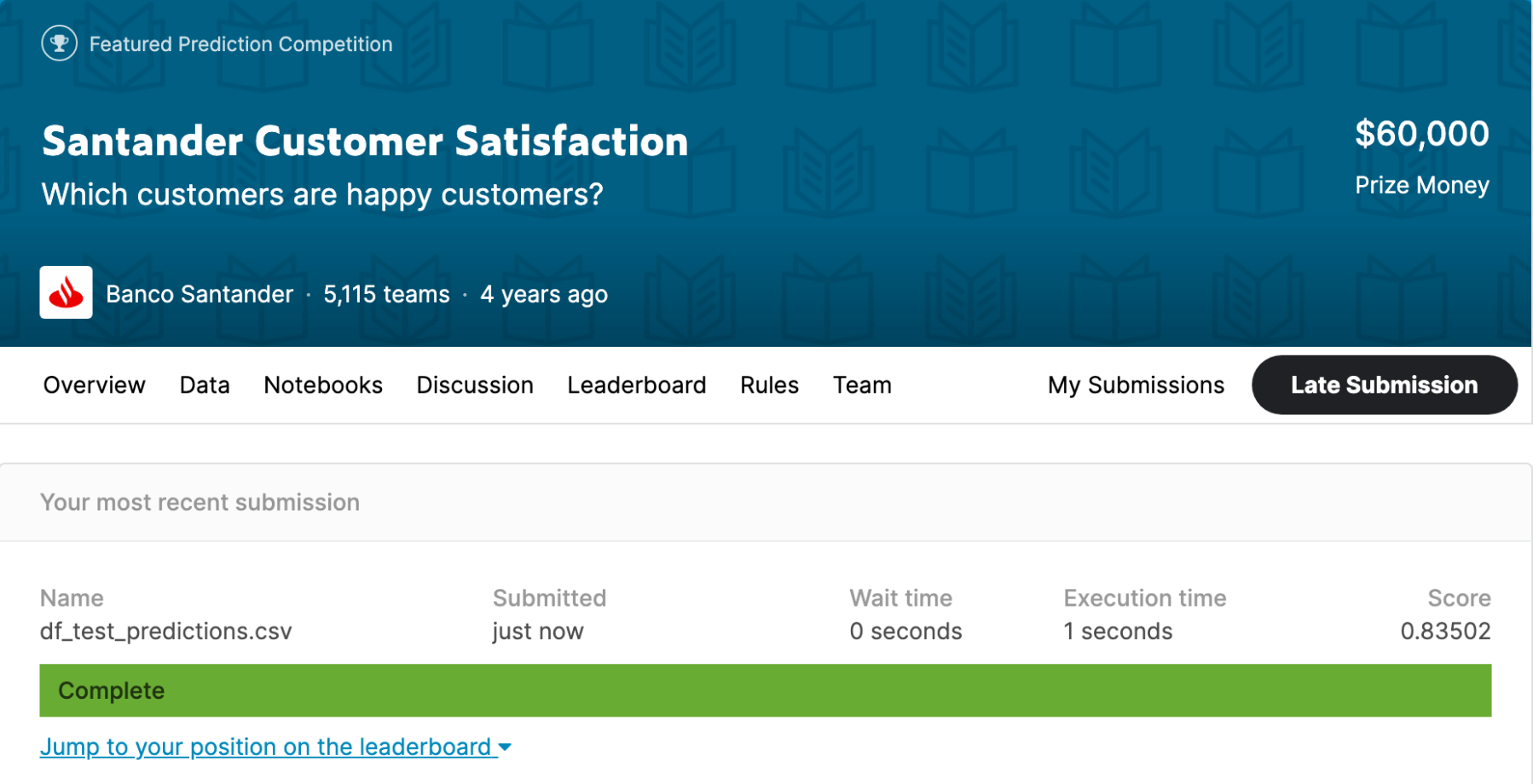Model AUC Score on Kaggle website.

It seems that our model also did a very good job at a dataset that it has never seen before. That is great!

# 7 Results Analysis

So, using the AUC metric, we arrived at a model that:

• On test data, split in step 3, scored 0.8477 for AUC;
• On Kaggle data, in 75818 new instances, scored 0,8305 for AUC.

It can therefore be concluded that the objective of creating a model that maximizes profits has been satisfactorily achieved.

In addition, we can analyze the ROC curve to better understand the AUC generated by the model. This brings a better understanding of how profit maximization can be done and we can see the optimum point for the classification decision threshold. Let’s plot it.

`# Code base on this post: https://stackoverflow.com/questions/25009284/how-to-plot-roc-curve-in-pythonimport sklearn.metrics as metrics# Calculate FPR and TPR for all thresholdsfpr, tpr, threshold = metrics.roc_curve(y_test, y_predicted)roc_auc = metrics.auc(fpr, tpr)# Plotting the ROC curveimport matplotlib.pyplot as pltfig, ax = plt.subplots(figsize = (20, 8))plt.title('Receiver Operating Characteristic', fontsize=18)plt.plot(fpr, tpr, 'b', label = 'AUC = %0.4f' % roc_auc)ax.spines['top'].set_visible(False)ax.spines['right'].set_visible(False)plt.legend(loc = 'upper left', fontsize = 16)plt.plot([0, 1], [0, 1],'r--')plt.xlim([0, 1])plt.ylim([0, 1])plt.ylabel('True Positive Rate', fontsize = 16)plt.xlabel('False Positive Rate', fontsize = 16)plt.show()`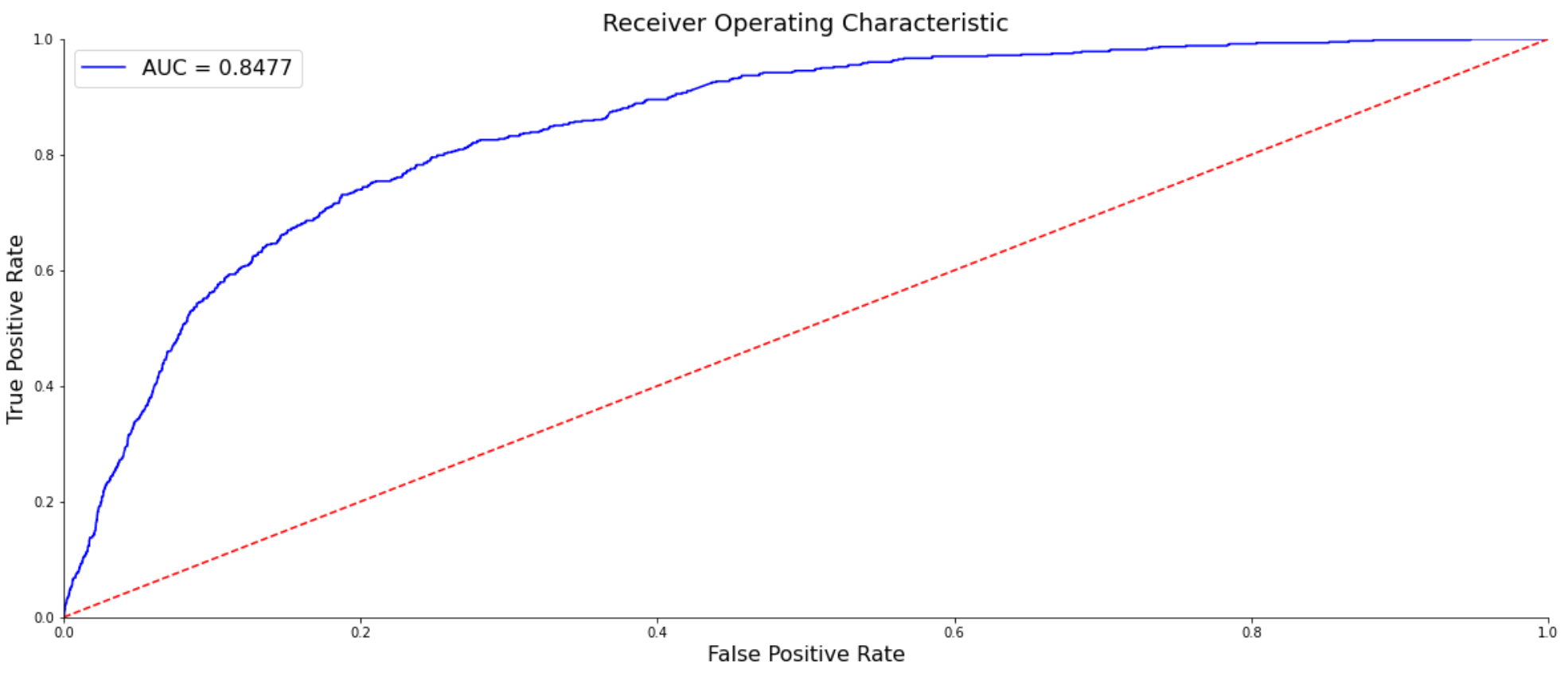ROC curve with the AUC for the model on X_test_selected (test data).

Finally, analyzing the ROC curve, we can choose the cut-off that maximizes profits. In this case, the point to be chosen is where the AUC curve approaches (shortest distance) the top of the y-axis. Thus, it can be concluded that the cut-off to be chosen is the one that generates an FPR of 0.12 and a TPR of approximately 0.65.

# 8 Next steps

• Work on feature engineering creating new features if possible;
• Try out different ML algorithms and compare them to the XGBClassifier
• As Caio Martins (https://github.com/CaioMar/) did and suggested me, a nice improvement would be to create a function that calculates the total profit. It is possible once we have values for TP and FP.

# 9 References

## The Startup

Get smarter at building your thing. Join The Startup’s +724K followers.

## The Startup

Get smarter at building your thing. Follow to join The Startup’s +8 million monthly readers & +724K followers.

Written by

## Pedro Couto

Data Science at Hella Gutmann (DE) linkedin.com/in/pedrocouto39/## The Startup

Get smarter at building your thing. Follow to join The Startup’s +8 million monthly readers & +724K followers.

## More From Medium

Medium is an open platform where 170 million readers come to find insightful and dynamic thinking. Here, expert and undiscovered voices alike dive into the heart of any topic and bring new ideas to the surface. Learn more

Follow the writers, publications, and topics that matter to you, and you’ll see them on your homepage and in your inbox. Explore

If you have a story to tell, knowledge to share, or a perspective to offer — welcome home. It’s easy and free to post your thinking on any topic. Write on Medium